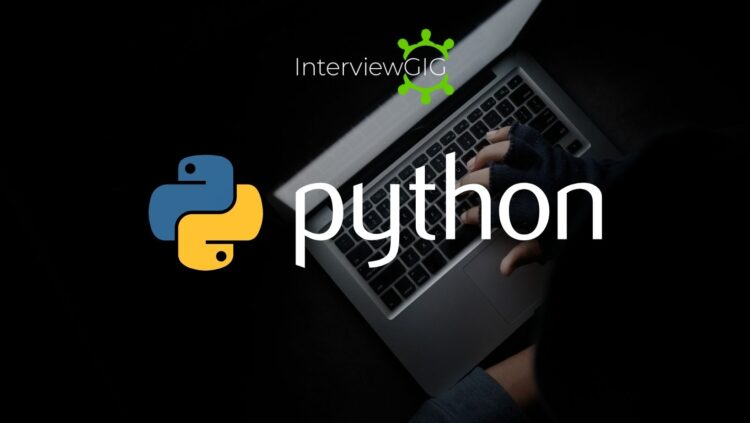# Python Program to Check Prime Number or Not

#### Python Program to Check Prime Number or Not

Write a Program to Check Prime Number or not.Prime Number in Mathematics:

In mathematics, a prime number is a natural number greater than 1 that has no positive divisors other than 1 and itself. In other words, a prime number is a number that cannot be evenly divided by any other number except 1 and itself.

For example, 2, 3, 5, 7, 11, and 13 are prime numbers.

Python Program to Check Prime Number:

Here’s a Python program that checks whether a given number is prime or not:

```num = int(input("Enter a number: ")) is_prime = True if num <= 1: is_prime = False else: for i in range(2, int(num ** 0.5) + 1): if num % i == 0: is_prime = False break print("Prime" if is_prime else "Not Prime")```

Explanation:

• The program starts by taking an integer input from the user using the input() function and converting it into an integer using the int() function. This input is stored in the variable num.
• The variable is_prime is initialized to True, assuming that the number is prime initially.
• The program uses conditional statements to handle different cases:
• If the input is less than or equal to 1, it’s not a prime number, so is_prime is set to False.
• Otherwise, a for loop is used to iterate from 2 to the square root of num (converted to an integer plus 1) using range(). This is done to optimize the loop by checking only up to the square root.
• Inside the loop, if num is divisible evenly by i (i.e., num % i equals 0), then the number is not prime, and is_prime is set to False. The loop is broken using the break statement.
• After the loop, the program prints “Prime” if is_prime is still True, indicating that the number is prime. Otherwise, it prints “Not Prime“.

Output:

```Enter a number: 17 Prime number```

In this example, if the user enters 17, the program checks whether 17 is divisible by any numbers from 2 to the square root of 17 (which is approximately 4). Since it’s not divisible by any of these numbers, the program determines that 17 is a prime number and displays “Prime” as the output.